Скачать презентацию Chi-square test or 2 test c What

c56853b8f86f3344b0de76f48a55b1f1.ppt

• Количество слайдов: 29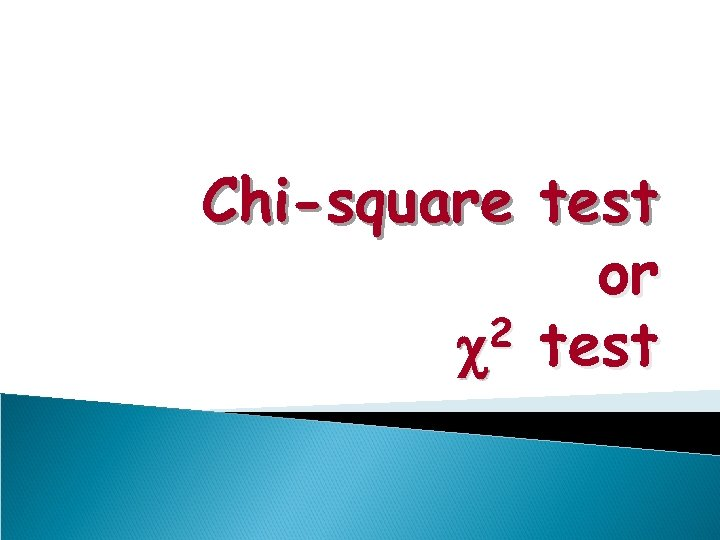Chi-square test or 2 test c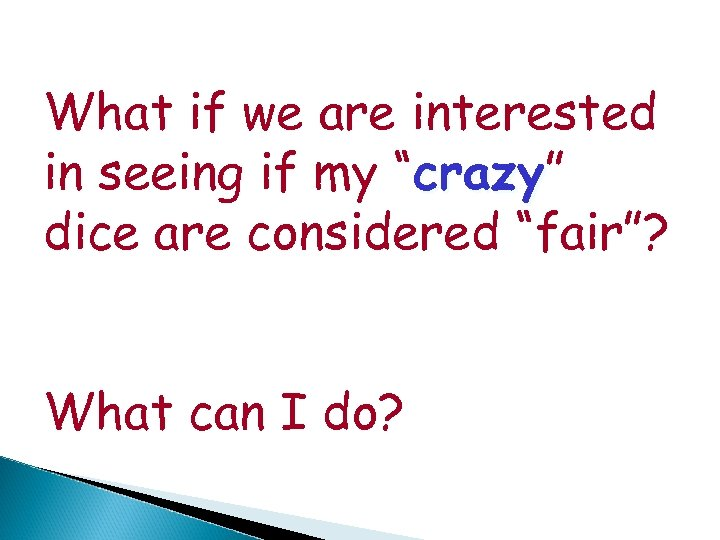What if we are interested in seeing if my “crazy” crazy dice are considered “fair”? What can I do?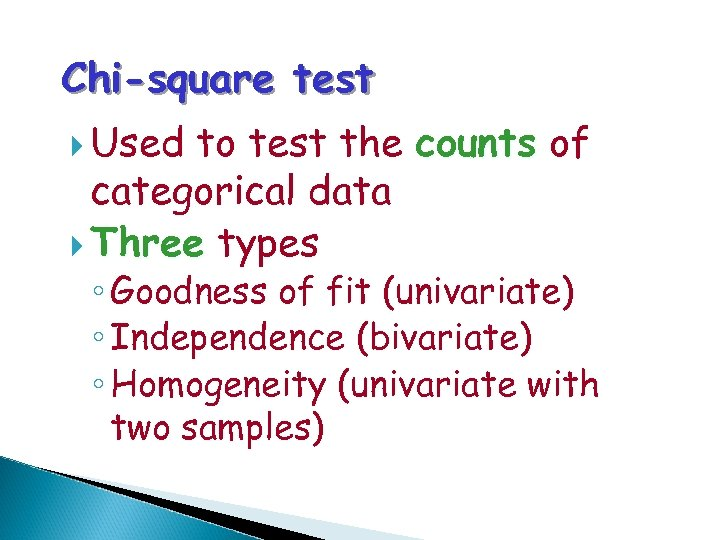Chi-square test Used to test the counts of categorical data Three types ◦ Goodness of fit (univariate) ◦ Independence (bivariate) ◦ Homogeneity (univariate with two samples)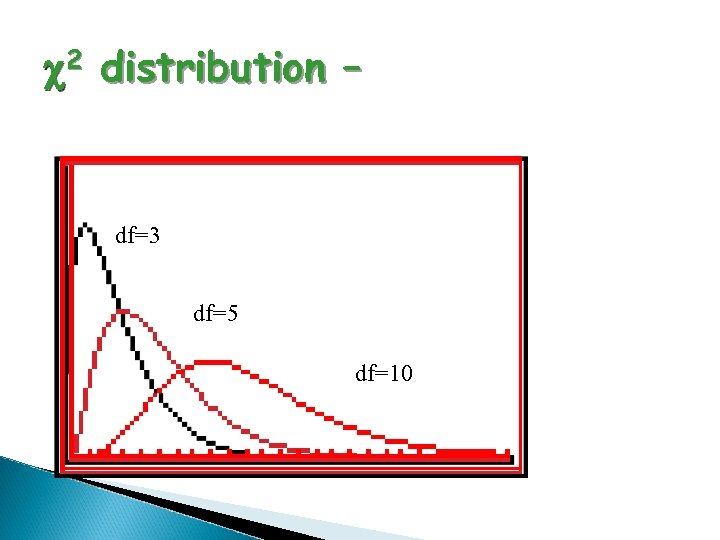c 2 distribution – df=3 df=5 df=10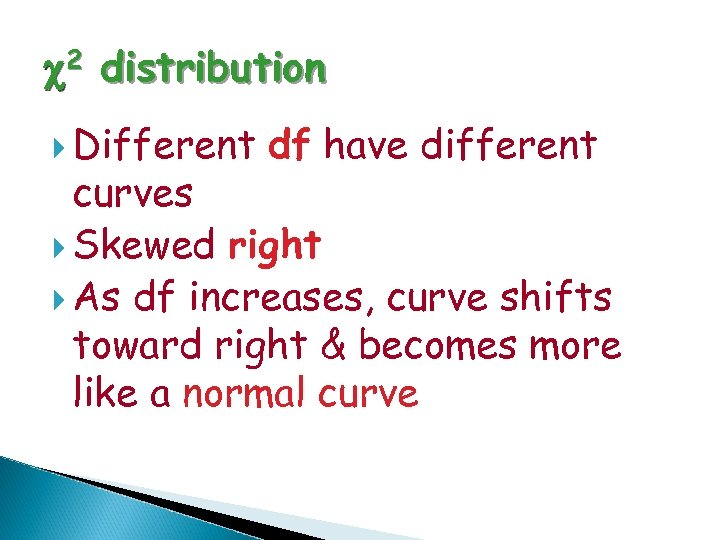c 2 distribution Different df have different curves Skewed right As df increases, curve shifts toward right & becomes more like a normal curve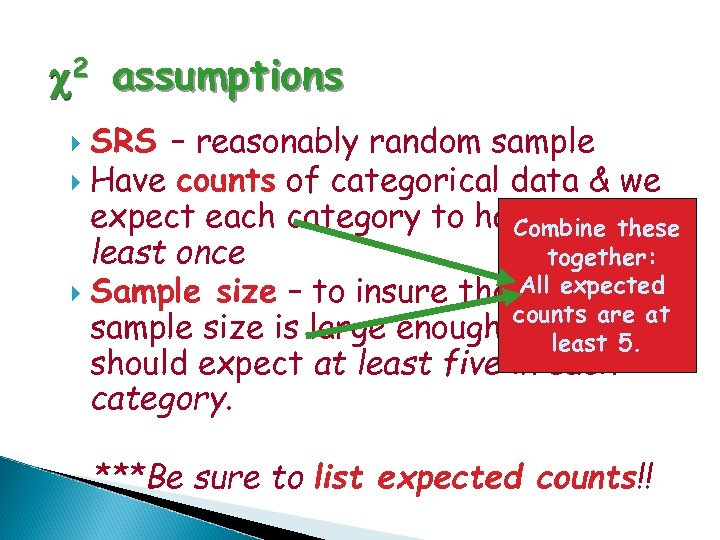c 2 assumptions SRS – reasonably random sample Have counts of categorical data & we expect each category to happen atthese Combine least once together: All expected Sample size – to insure that the counts are at sample size is large enough weleast 5. should expect at least five in each category. ***Be sure to list expected counts!!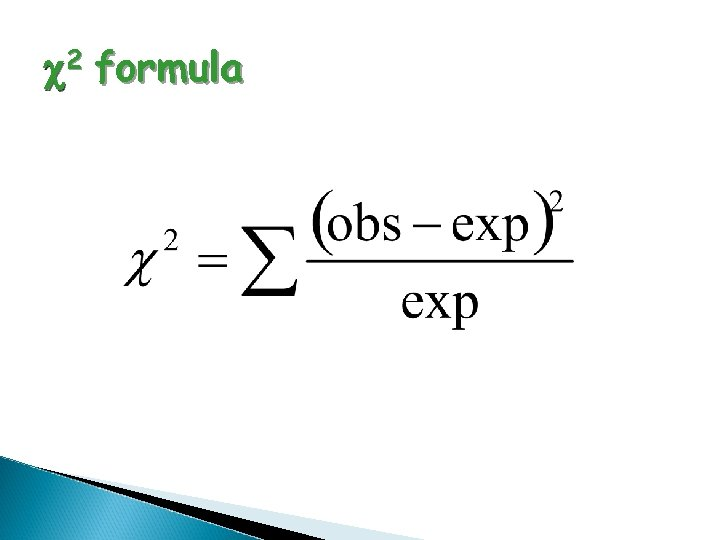c 2 formula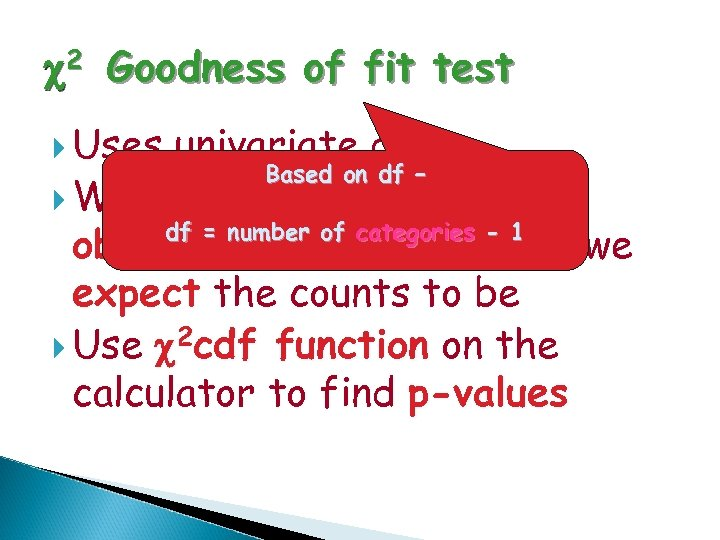c 2 Goodness of fit test Uses univariate data Based on df – Want to see how well the df = number of categories - 1 observed counts “fit” what we expect the counts to be Use c 2 cdf function on the calculator to find p-values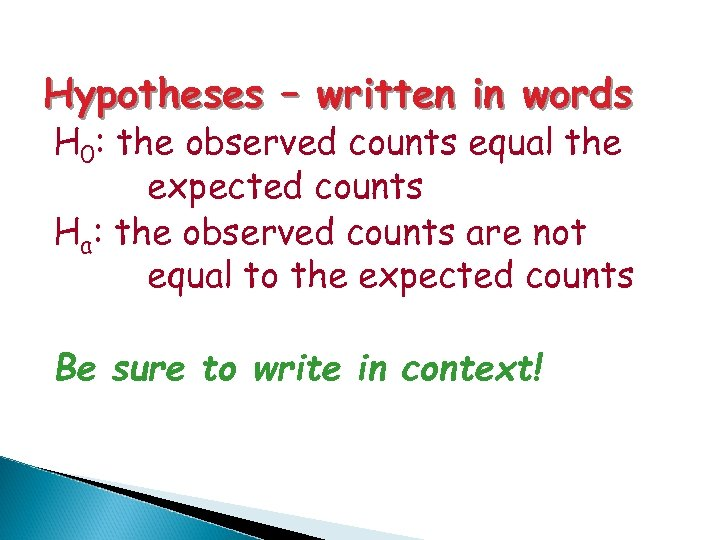Hypotheses – written in words H 0: the observed counts equal the expected counts Ha: the observed counts are not equal to the expected counts Be sure to write in context!Let’s test our dice!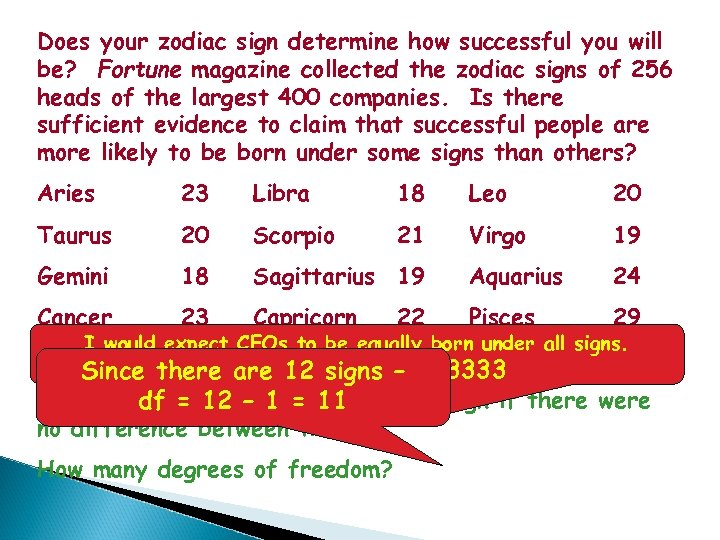Does your zodiac sign determine how successful you will be? Fortune magazine collected the zodiac signs of 256 heads of the largest 400 companies. Is there sufficient evidence to claim that successful people are more likely to be born under some signs than others? Aries 23 Libra 18 Leo 20 Taurus 20 Scorpio 21 Virgo 19 Gemini 18 Sagittarius 19 Aquarius 24 Cancer 23 Capricorn Pisces 29 22 I would expect CEOs to be equally born under all signs. So 256/12 = 21. 333333 Since there are 12 signs – How many would you 1 = 11 in each sign if there were df = 12 – expect no difference between them? How many degrees of freedom?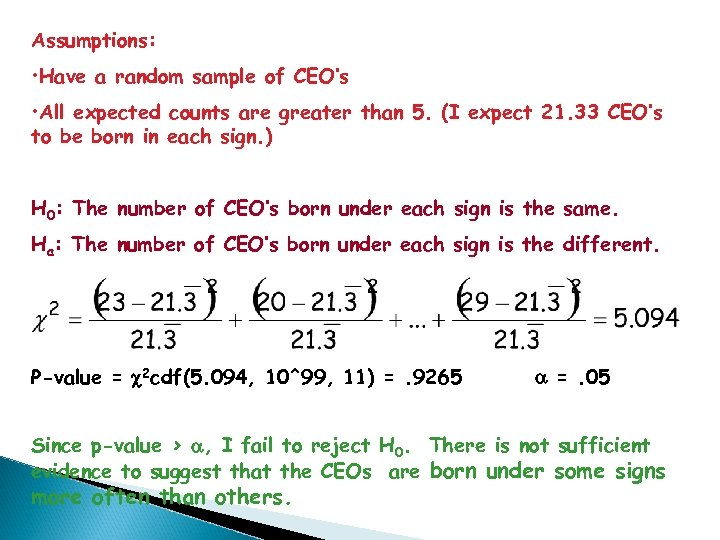Assumptions: • Have a random sample of CEO’s • All expected counts are greater than 5. (I expect 21. 33 CEO’s to be born in each sign. ) H 0: The number of CEO’s born under each sign is the same. Ha: The number of CEO’s born under each sign is the different. P-value = c 2 cdf(5. 094, 10^99, 11) =. 9265 a =. 05 Since p-value > a, I fail to reject H 0. There is not sufficient evidence to suggest that the CEOs are born under some signs more often than others.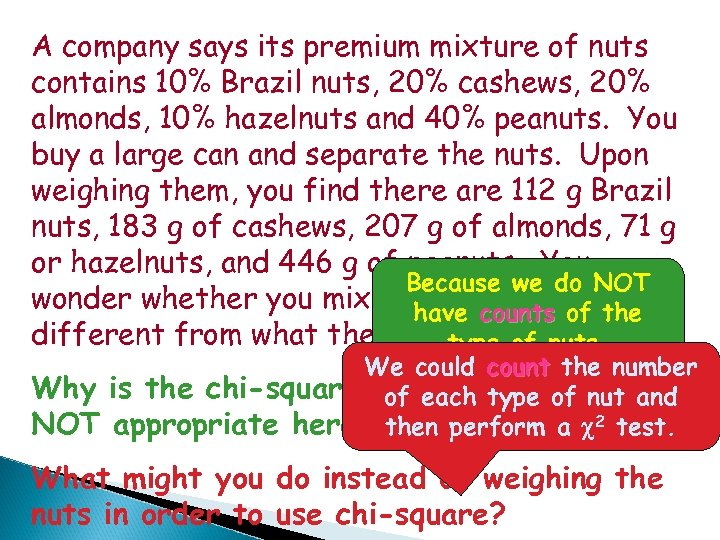A company says its premium mixture of nuts contains 10% Brazil nuts, 20% cashews, 20% almonds, 10% hazelnuts and 40% peanuts. You buy a large can and separate the nuts. Upon weighing them, you find there are 112 g Brazil nuts, 183 g of cashews, 207 g of almonds, 71 g or hazelnuts, and 446 g of peanuts. You Because we do NOT wonder whether you mix is significantly the have counts of different from what the company advertises? type of nuts. Why NOT We could count the number is the chi-square goodness-of-fit test of each type of nut and appropriate here? then perform a c 2 test. What might you do instead of weighing the nuts in order to use chi-square?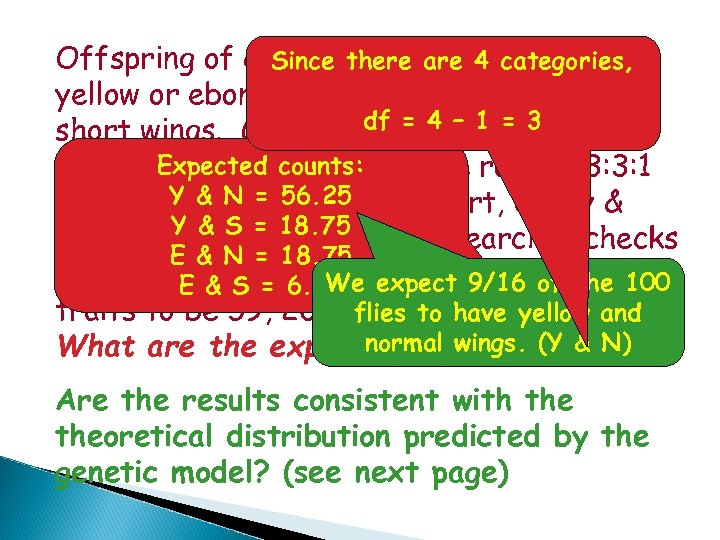Offspring of certain there are 4 categories, Since fruit flies may have yellow or ebony bodies and normal wings or df = 4 1 = 3 short wings. Genetic theory –predicts that Expected appear these traits willcounts: in the ratio 9: 3: 3: 1 Y & N = 56. 25 (yellow & normal, yellow & short, ebony & Y & S = 18. 75 normal, ebony & short) A researcher checks E & N = 18. 75 100 such E & S and finds the distribution 100 flies = 6. 25 expect 9/16 of the of We traits to be 59, 20, 11, and 10, respectively. flies to have yellow and normal wings. df? What are the expected counts? (Y & N) Are the results consistent with theoretical distribution predicted by the genetic model? (see next page)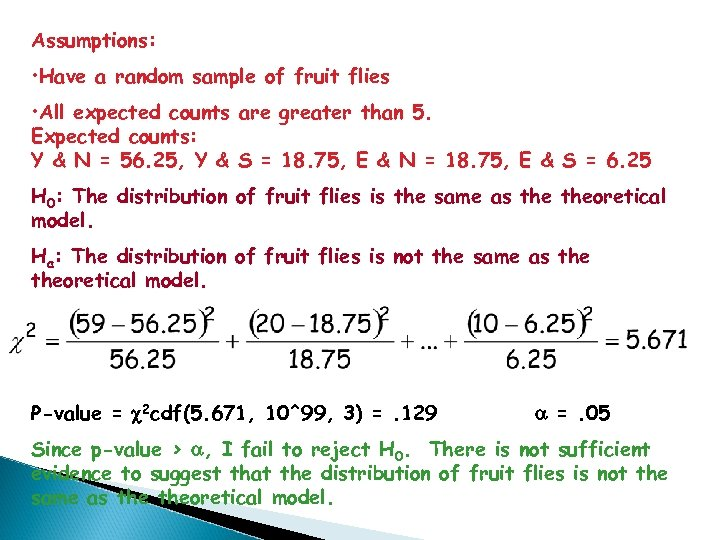Assumptions: • Have a random sample of fruit flies • All expected counts are greater than 5. Expected counts: Y & N = 56. 25, Y & S = 18. 75, E & N = 18. 75, E & S = 6. 25 H 0: The distribution of fruit flies is the same as theoretical model. Ha: The distribution of fruit flies is not the same as theoretical model. P-value = c 2 cdf(5. 671, 10^99, 3) =. 129 a =. 05 Since p-value > a, I fail to reject H 0. There is not sufficient evidence to suggest that the distribution of fruit flies is not the same as theoretical model.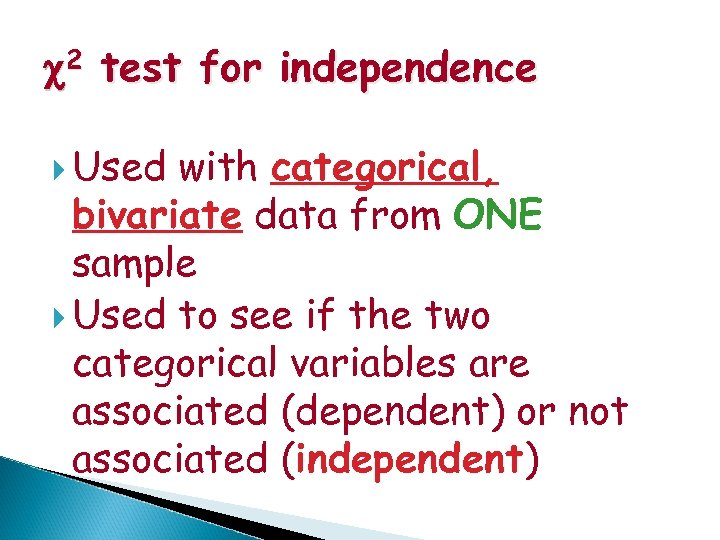c 2 test for independence Used with categorical, bivariate data from ONE sample Used to see if the two categorical variables are associated (dependent) or not associated (independent)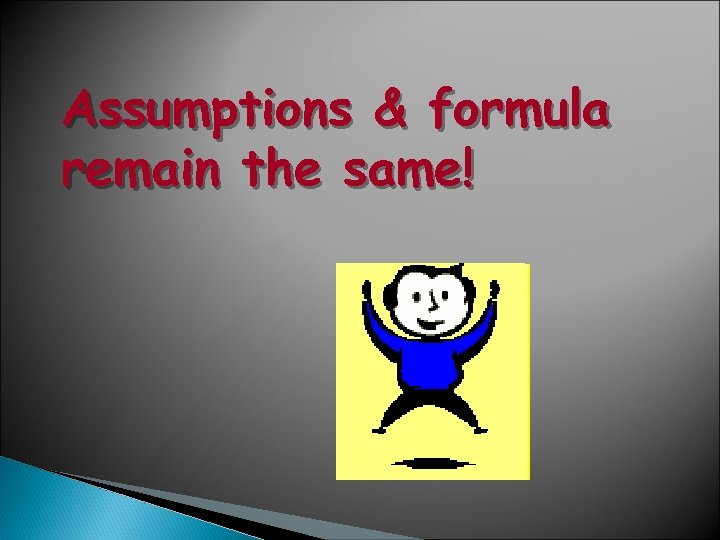Assumptions & formula remain the same!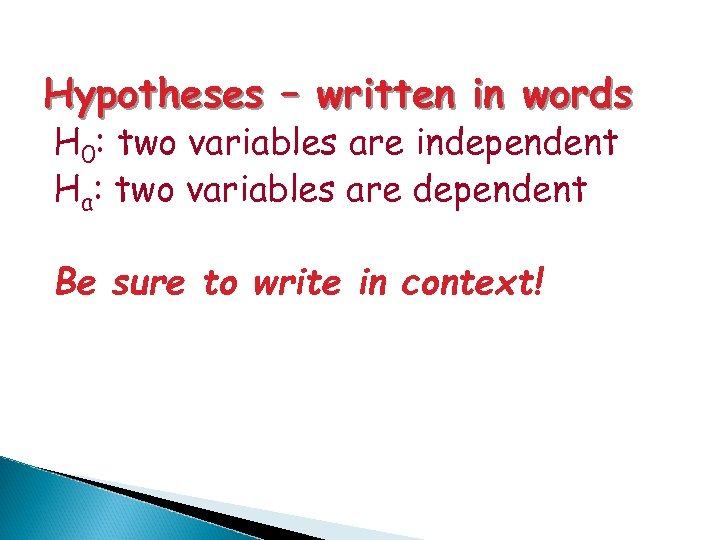Hypotheses – written in words H 0: two variables are independent Ha: two variables are dependent Be sure to write in context!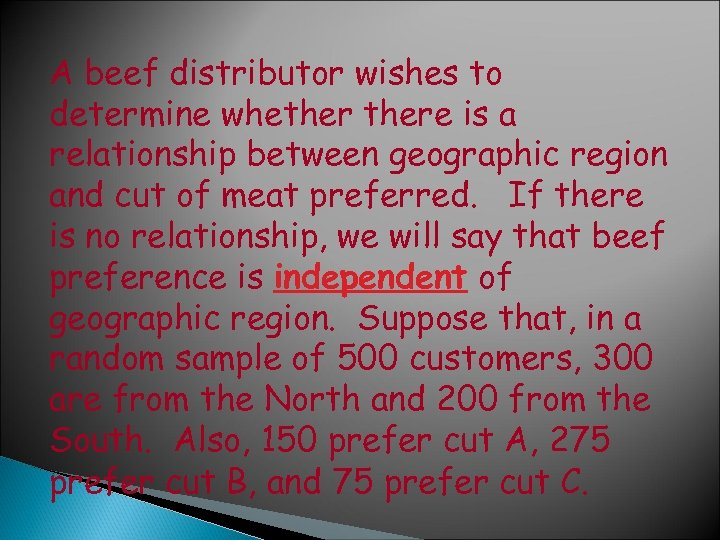A beef distributor wishes to determine whethere is a relationship between geographic region and cut of meat preferred. If there is no relationship, we will say that beef preference is independent of geographic region. Suppose that, in a random sample of 500 customers, 300 are from the North and 200 from the South. Also, 150 prefer cut A, 275 prefer cut B, and 75 prefer cut C.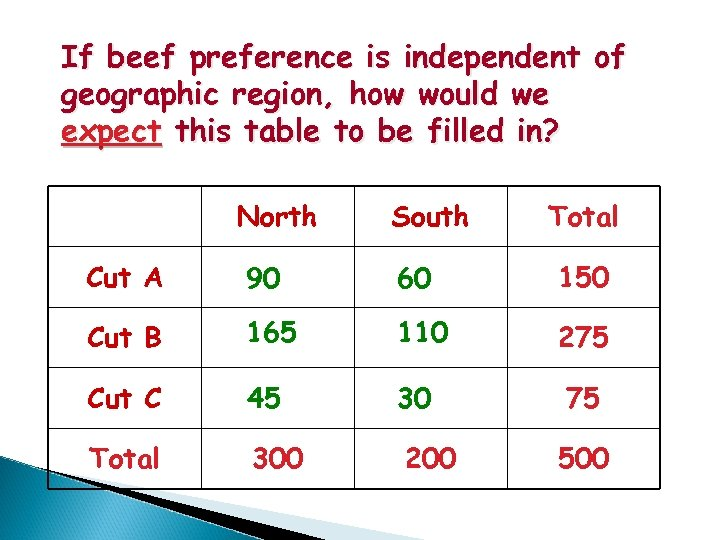If beef preference is independent of geographic region, how would we expect this table to be filled in? North South Total Cut A 90 60 150 Cut B 165 110 275 Cut C 45 30 Total 300 200 75 500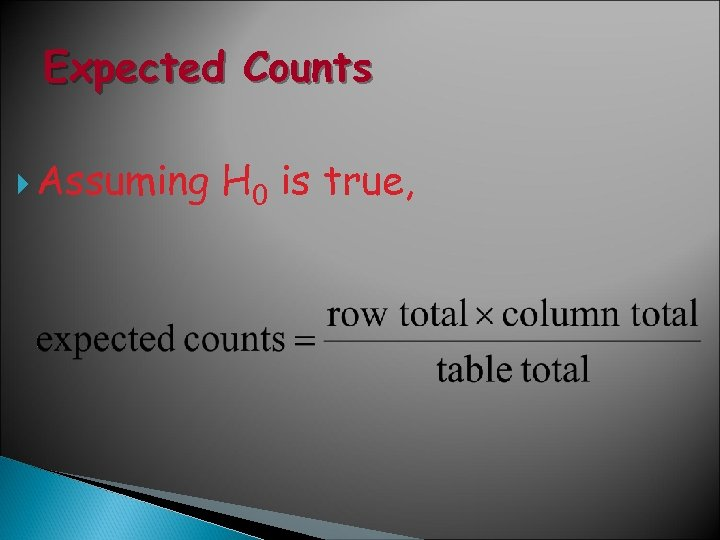Expected Counts Assuming H 0 is true,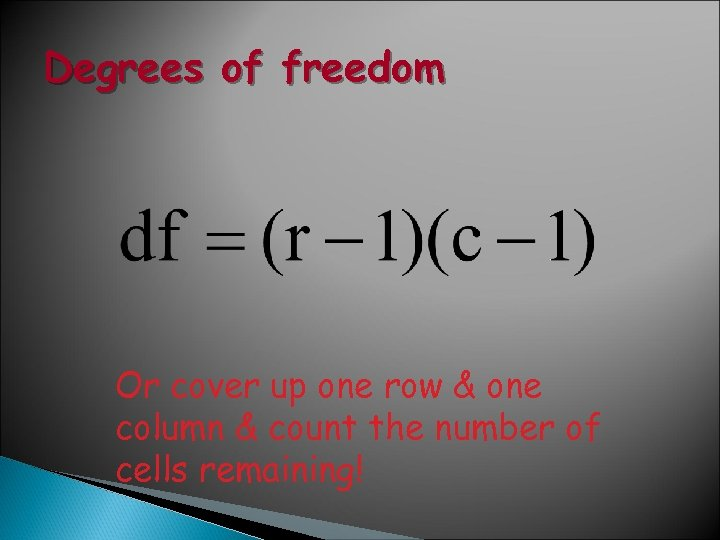Degrees of freedom Or cover up one row & one column & count the number of cells remaining!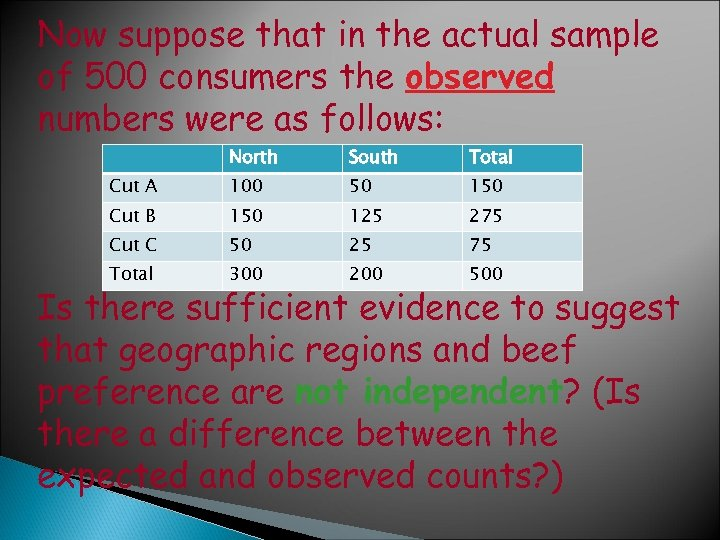Now suppose that in the actual sample of 500 consumers the observed numbers were as follows: North South Total Cut A 100 50 150 Cut B 150 125 275 Cut C 50 25 75 Total 300 200 500 Is there sufficient evidence to suggest that geographic regions and beef preference are not independent? (Is there a difference between the expected and observed counts? )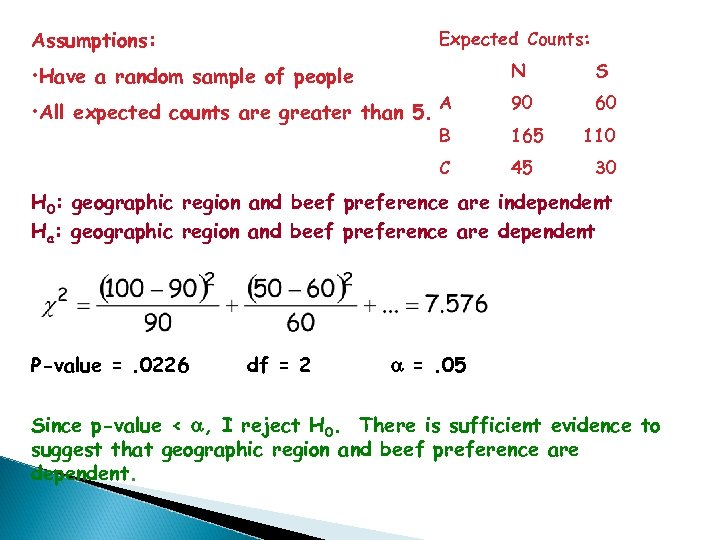Assumptions: Expected Counts: • Have a random sample of people N S • All expected counts are greater than 5. A 90 60 B 165 C 45 110 30 H 0: geographic region and beef preference are independent Ha: geographic region and beef preference are dependent P-value =. 0226 df = 2 a =. 05 Since p-value < a, I reject H 0. There is sufficient evidence to suggest that geographic region and beef preference are dependent.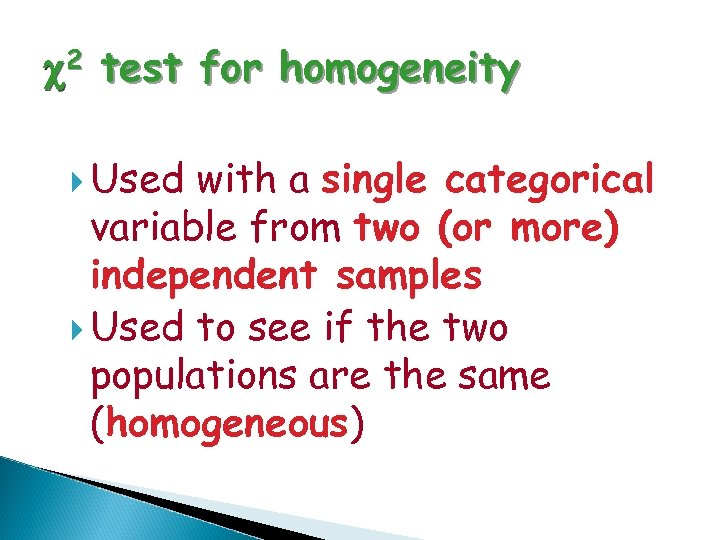c 2 test for homogeneity Used with a single categorical variable from two (or more) independent samples Used to see if the two populations are the same (homogeneous)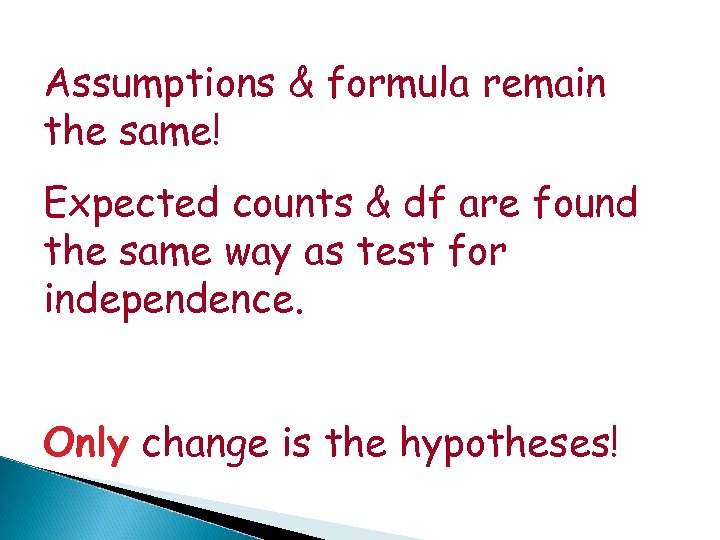Assumptions & formula remain the same! Expected counts & df are found the same way as test for independence. Only change is the hypotheses!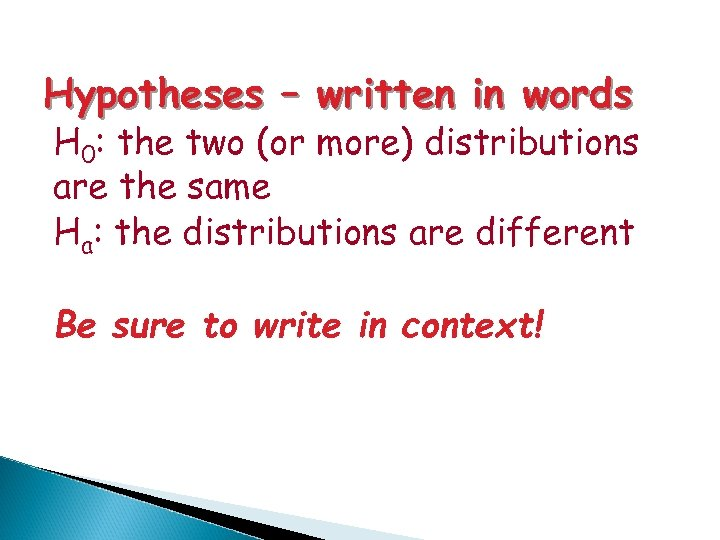Hypotheses – written in words H 0: the two (or more) distributions are the same Ha: the distributions are different Be sure to write in context!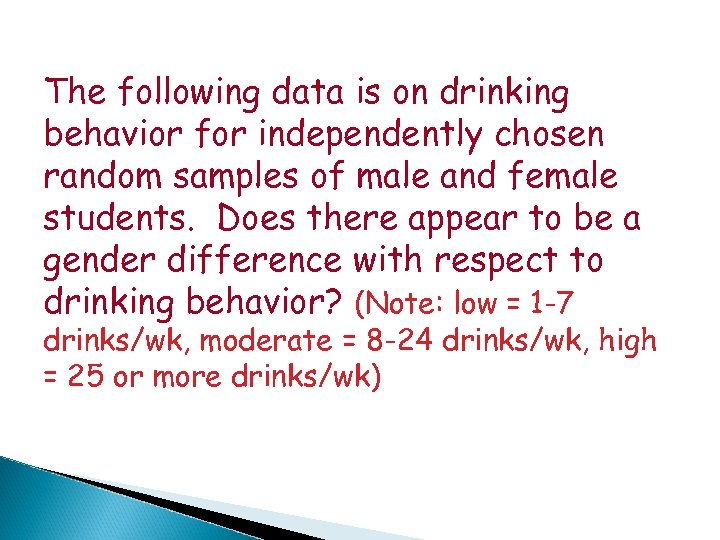The following data is on drinking behavior for independently chosen random samples of male and female students. Does there appear to be a gender difference with respect to drinking behavior? (Note: low = 1 -7 drinks/wk, moderate = 8 -24 drinks/wk, high = 25 or more drinks/wk)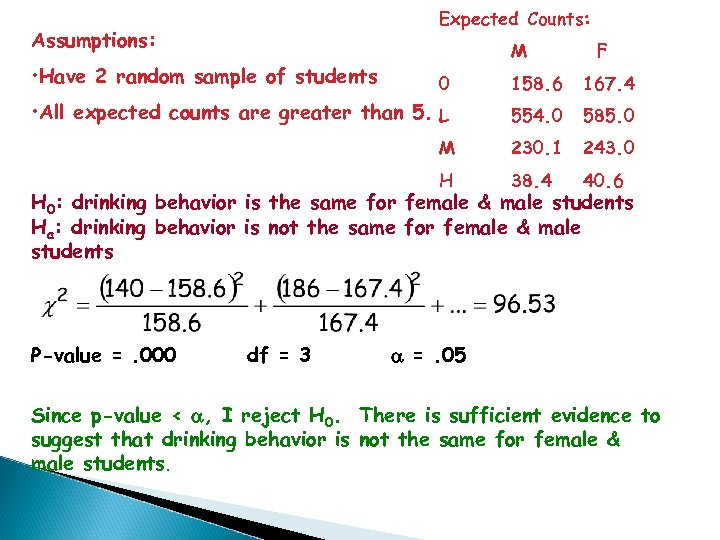Expected Counts: Assumptions: • Have 2 random sample of students M F 0 158. 6 167. 4 • All expected counts are greater than 5. L 554. 0 585. 0 M 230. 1 243. 0 H 38. 4 40. 6 H 0: drinking behavior is the same for female & male students Ha: drinking behavior is not the same for female & male students P-value =. 000 df = 3 a =. 05 Since p-value < a, I reject H 0. There is sufficient evidence to suggest that drinking behavior is not the same for female & male students.